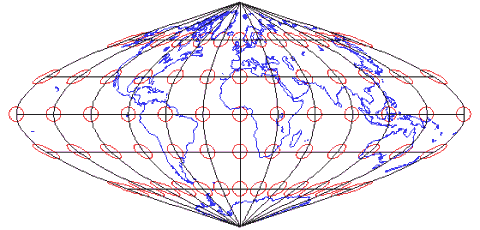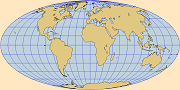# Hans Havlicek: Mathematical Cartography

This is a series of lectures and exercises for students of Mathematics or Descriptive Geometry. We present the mathematical background that is used when mapping the (round) surface of the earth to a (flat) plane.The earth: Nov. 11th, 2000 at 16:00 UTC

## The Earth and Mathematics

For the purposes of geography it is usually sufficient to assume that the earth is a sphere; this point of view is also adopted in our lectures.

In geodesy, however, the oblateness of the earth at the poles has to be taken into account. So an ellipsoid of revolution is taken as a mathematical model of the earth.

Neither a sphere nor an ellipsoid can be mapped in a plane without distortion. The theory of distortion is a central part of these lectures on mathematical cartography.

It seems that most people are not aware of the fact that the maps in their atlases are based upon a discipline of higher mathematics, called differential geometry.

## Course

### 1. Introduction

• Mathematical models of the earth
• Earth and sun: tropics and polar circles
• Spherical coordinates (longitudinal and latitudinal circles)
• Basic differential geometry of curves and surfacesEquidistant projection on a cylinder

### 2. Conical Projections

• Conical, azimuthal, and cylindrical projections
• Equidistant projections
• The theory of distortion I: Distances (Tissot's indicatrix)
• Conformal projections in general
• Conformal conical projections
• Stereographic projection
• Mercator's conformal cylindrical projection
• The theory of distortion II: Angles
• Equal-area projections in general
• Lambert's equal-area conical, azimuthal and cylindrical projections
• The theory of distortion III: Areas
• Perspective projections in general
• Gnomonic projection

### 3. Pseudoconical Projections

• Pseudoconical and pseudocylindrical projections
• Bonne, Stab-Werner, and Sanson
• Mollweide's projection
• Modifying a map projection
• Affine linear combination of maps: Eckert V (pseudocylindrical)

### 4. The Aspect

• Normal aspect
• Transverse aspect
• Oblique aspect
• Transformation formulas.

## Lecture Notes

Lecture notes (Formelsammlung Kartenentwürfe, mit zahlreichen Illustrationen / Formulas for Map Projections, in German, with many illustrations) can be downloaded from my page on Publications.

## Illustrations

All map projections that are exhibited in these lectures (and many more) can be found in our Picture Gallery of Map Projections.The following material has been prepared in part by one of my students, Harald Veronik, with the programme 'Maple V'.

From North to South. This sequence of images starts with the equidistant azimuthal projection onto the tangent plane at the north pole. This is gradually changed to equidistant conical projections with the standard parallel moving towards the equator. So we arrive at the equidistant cylindrical projection. As the standard parallel moves further to the south, we finally obtain the equidistant azimuthal projection onto the tangent plane in the south pole.

Tissot's Indicatrix. An easy way of visualising the distortion of a map at a given point P is Tissot's indicatrix, which is an ellipse centred at P. If the indicatrix is a circle then the map is conformal (angle preserving) at P, otherwise the main axes of the ellipse give the directions of maximal and minimal distortion.

In our example you can see indicatrices for Sanson's projection. Here the image is undistorted (up to the scale) along the equator and the meridian 0° (Greenwich). It is worth noting that all indicatrices, though they of different shape, have the same area. This illustrates the fact that Sanson's projection is area preserving.Picture Gallery of Map Projections

Aspect of a Projection
H. Havlicek: Publications
H. Havlicek: Lineare Algebra für Technische Mathematik
(in German)

H. Havlicek: Evaluation of Teaching
Teaching
Sitemap

## PosterLehrveranstaltung Kartenentwürfe
(PDF - in German)